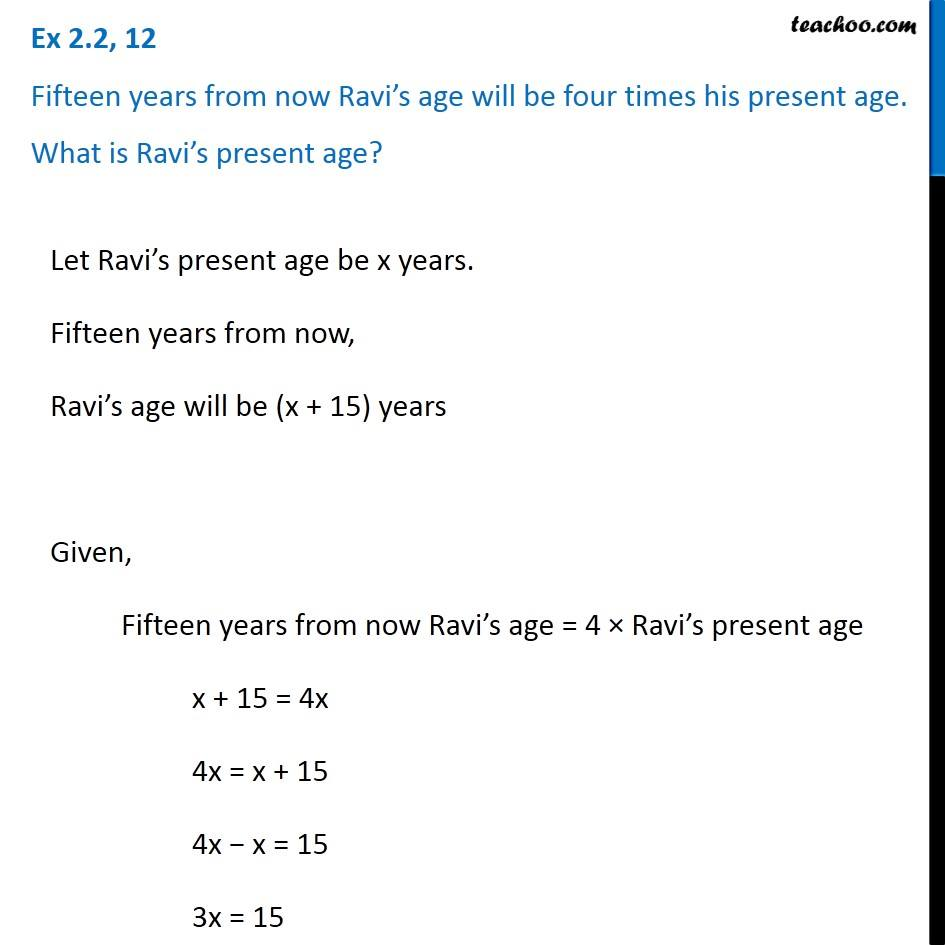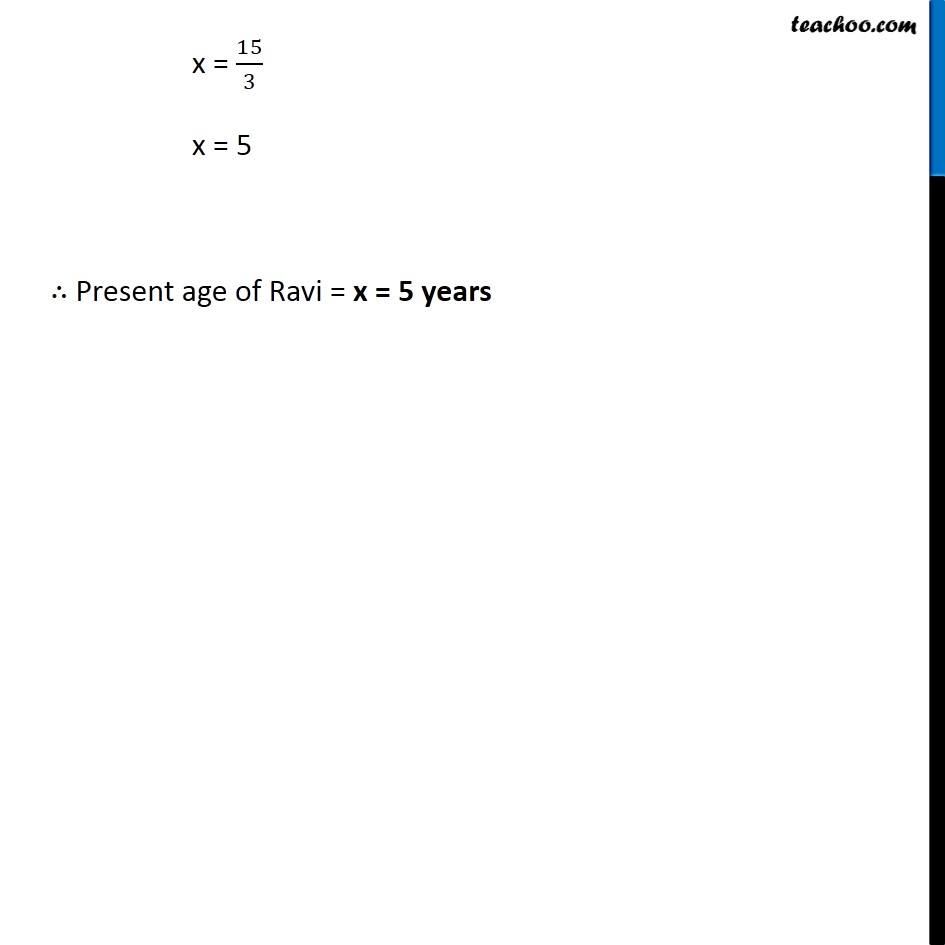Solving easy equations - Word Problems

Chapter 2 Class 8 Linear Equations in One Variable
Serial order wiseLearn in your speed, with individual attention - Teachoo Maths 1-on-1 Class

### Transcript

Question 12 Fifteen years from now Ravi’s age will be four times his present age. What is Ravi’s present age?Let Ravi’s present age be x years. Fifteen years from now, Ravi’s age will be (x + 15) years Given, Fifteen years from now Ravi’s age = 4 × Ravi’s present age x + 15 = 4x 4x = x + 15 4x − x = 15 3x = 15 x = 15/3 x = 5 ∴ Present age of Ravi = x = 5 years